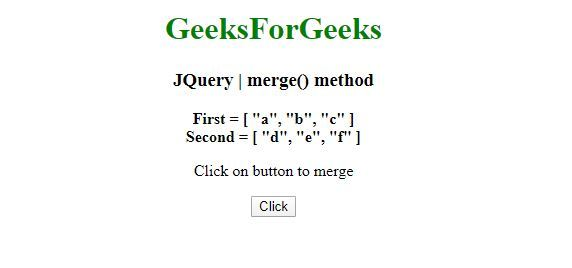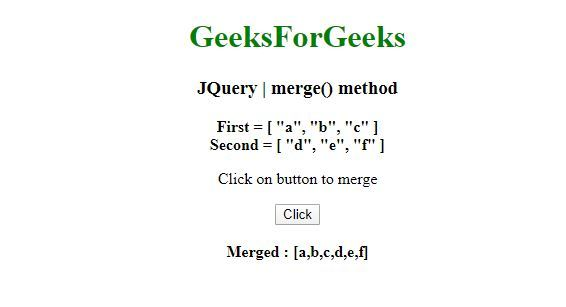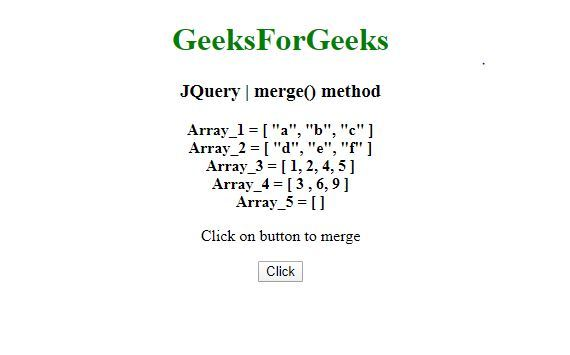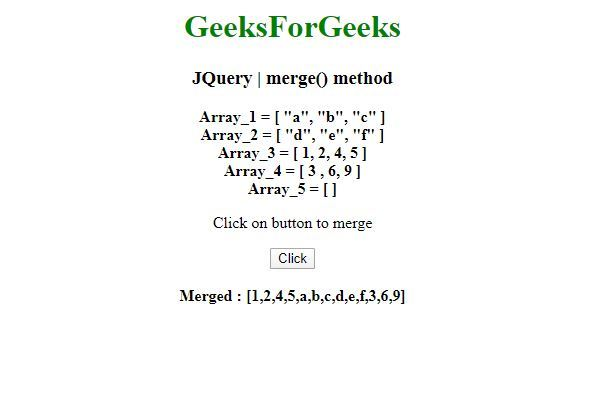# JQuery | merge() method

This merge() Method in jQuery is used to merge the contents of two arrays together into the first array.

Syntax:

```jQuery.merge( first, second )
```

Parameters: The merge() method accepts only one parameter that is mentioned above and described below:

• first : This parameter is the first array-like object to merge, the elements of second added.
• second : This parameter is the second array-like object to merge into the first, unaltered.
• Return Value: It returns the merged array.

Example 1: In this example, the merge() Method merges only two arrays .

 ` ` `<``html``> ` `<``head``> ` `<``meta` `charset``=``"utf-8"``> ` `<``title``>JQuery | merge() method  ` `<``script` `src``=``"https://code.jquery.com/jquery-3.4.1.js"``> ` ` `  ` ` `<``body` `style``=``"text-align:center;"``>  ` `     `  `    ``<``h1` `style``=``"color: green"``>  ` `        ``GeeksForGeeks  ` `    ``  ` `     `  `    ``<``h3``>JQuery | merge() method ` `     `  `    ``<``b``>First = [ "a", "b", "c" ]<``br``> ` `    ``<``b``>Second = [ "d", "e", "f" ] ` `    ``<``br``> ` `    ``<``p``>Click on button to merge ` `    ``<``button` `onclick``=``"geek()"``>Click ` `    ``<``br``><``br``> ` `    ``<``b` `id``=``"geeks"``> ` `     `  `    ``<``script``> ` `    ``function geek() { ` `        ``var first = [ "a", "b", "c" ]; ` `        ``var second = [ "d", "e", "f" ]; ` `        ``var n = \$.merge(first, second ); ` `        ``document.getElementById("geeks").innerHTML = "Merged : [" + n + "]"; ` `    ``} ` `    `` ` ` ` `                                                                                                     `

Output:
Before Click:After Click:Example 2: In this example, the merge() Method merges more than two array.

 ` ` `<``html``> ` `<``head``> ` `<``meta` `charset``=``"utf-8"``> ` `<``title``>JQuery | merge() method  ` `<``script` `src``=``"https://code.jquery.com/jquery-3.4.1.js"``> ` ` `  ` ` `<``body` `style``=``"text-align:center;"``>  ` `     `  `    ``<``h1` `style``=``"color: green"``>  ` `        ``GeeksForGeeks  ` `    ``  ` `     `  `    ``<``h3``>JQuery | merge() method ` `     `  `    ``<``b``>Array_1 = [ "a", "b", "c" ]<``br``> ` `    ``<``b``>Array_2 = [ "d", "e", "f" ]    <``br``> ` `    ``<``b``>Array_3 = [ 1, 2, 4, 5 ]    <``br``> ` `    ``<``b``>Array_4 = [ 3, 6, 9 ]<``br``> ` `    ``<``b``>Array_5 = [ ] ` `    ``<``br``> ` `    ``<``p``>Click on button to merge ` `    ``<``button` `onclick``=``"geek()"``>Click ` `    ``<``br``><``br``> ` `    ``<``b` `id``=``"geeks"``> ` `     `  `    ``<``script``> ` `    ``function geek() { ` `        ``var fi = [ "a", "b", "c" ]; ` `        ``var se = [ "d", "e", "f" ]; ` `        ``var th = [ 1, 2, 4, 5 ]; ` `        ``var fo = [ 3, 6, 9 ]; ` `        ``var fif = []; ` `        ``var n = \$.merge( ` `th, \$.merge(\$.merge(fif, \$.merge(fi, se )), fo)); ` ` `  `        ``document.getElementById( ` `"geeks").innerHTML = "Merged : [" + n + "]"; ` `    ``} ` `    `` ` ` ` `                                                 `

Output:
Before Click:After Click:My Personal Notes arrow_drop_upCheck out this Author's contributed articles.

If you like GeeksforGeeks and would like to contribute, you can also write an article using contribute.geeksforgeeks.org or mail your article to contribute@geeksforgeeks.org. See your article appearing on the GeeksforGeeks main page and help other Geeks.

Please Improve this article if you find anything incorrect by clicking on the "Improve Article" button below.

Article Tags :

Be the First to upvote.

Please write to us at contribute@geeksforgeeks.org to report any issue with the above content.